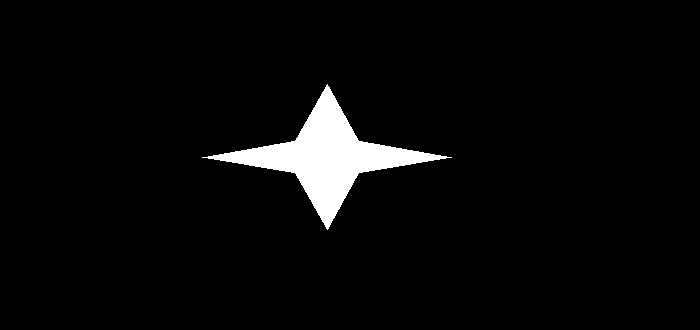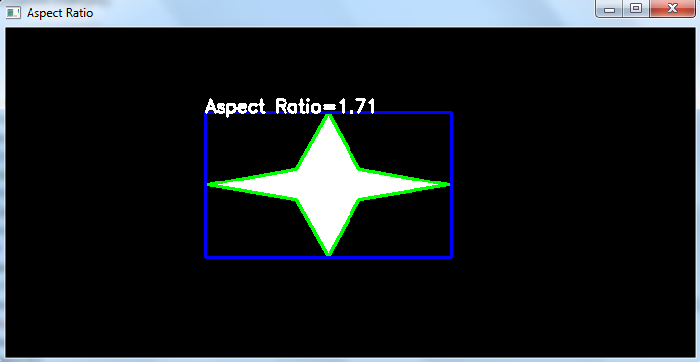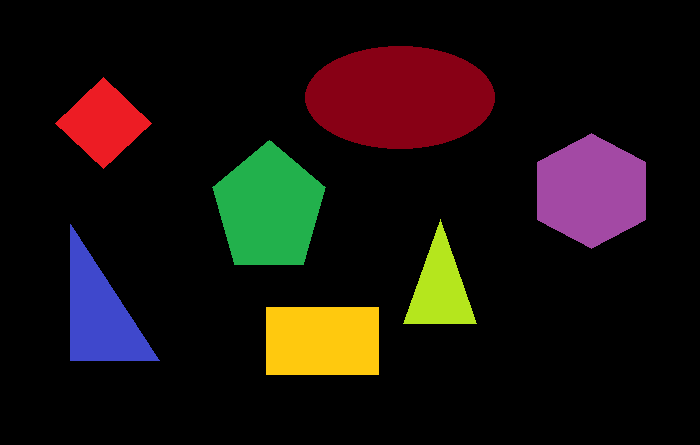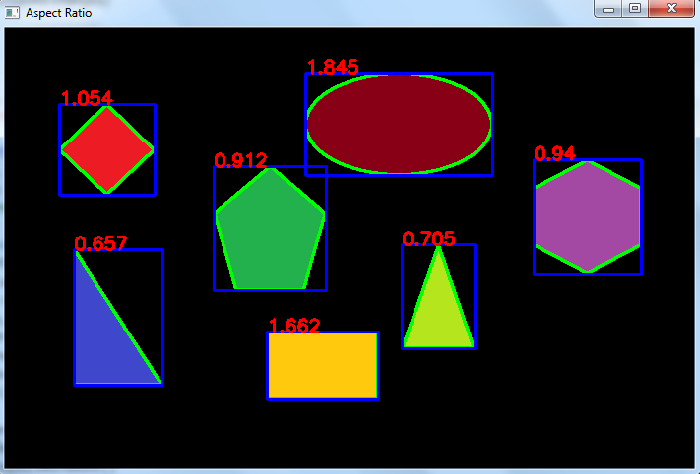# How to compute the aspect ratio of an object in an image using OpenCV Python?

The aspect ratio of an object is computed as the ratio between the width and height of the bounding rectangle of the object. So, to compute the aspect ratio, we first have to find the bounding rectangle of the object. Bounding rectangle of an object can be found using cv2.boundingRect() function.

It accepts the contour points of the object and returns top-left coordinate (x,y) and (width, height) of the bounding rectangle. We use the width and height to compute the aspect ratio.

## Syntax

x, y, w, h = cv2.boundingRect(cnt)
aspect_ratio = float(w)/h


Here, "cnt" is a numpy array of the contour points of an object in the image.

## Steps

You can use the following steps to compute aspect ratio of an object in an image −

Import the required library. In all the following Python examples, the required Python library is OpenCV. Make sure you have already installed it.

import cv2


img = cv2.imread('fourpoint-star.png')
gray = cv2.cvtColor(img, cv2.COLOR_BGR2GRAY)


Apply thresholding on the grayscale image to create a binary image. Adjust the second argument for better detection of contour.

ret,thresh = cv2.threshold(gray,150,255,0)


Find the contours in the image using cv2.findContours() function.

contours, _ = cv2.findContours(thresh, cv2.RETR_TREE, cv2.CHAIN_APPROX_SIMPLE)


Select a contour cnt or loop over all contours. Compute the aspect ratio using width and height of the bounding rectangle of the object.

cnt = contours
x, y, w, h = cv2.boundingRect(cnt)
ar = float(w)/h


Optionally you can draw the contours and bounding rectangle on the input image. Also put the aspect ratio as text on the image

cv2.drawContours(img, [cnt], -1, (0,255,255), 3)
cv2.rectangle(img,(x,y),(x+w,y+h),(255,0,0),2)


Print the aspect ratio and display the image with the drawn contours, bounding rectangle.

print("Aspect Ratio of object: ", ar)
cv2.imshow("Aspect Ratio", img)
cv2.waitKey(0)
cv2.destroyAllWindows()


Let's have a look at some examples for more clear understanding.

## Example 1

In this Python program, we compute the aspect ratio of an object in the image. We draw the contour and bounding rectangle for the object on the image. We also put the aspect ratio as text for the object.

# import required libraries
import cv2

# convert the image to grayscale
gray = cv2.cvtColor(img, cv2.COLOR_BGR2GRAY)

# apply thresholding to convert grayscale to binary image
ret,thresh = cv2.threshold(gray,150,255,0)

# find the contours
contours,hierarchy = cv2.findContours(thresh, cv2.RETR_TREE,cv2.CHAIN_APPROX_SIMPLE)
print("Number of objects detected:", len(contours))

# define function to compute aspect ratio
def aspect_ratio(cnt):
x, y, w, h = cv2.boundingRect(cnt)
ratio = float(w)/h
return ratio

# select first contour
cnt = contours

# find the aspect ratio
ar = aspect_ratio(cnt)

# round it to two decimal points
ar = round(ar, 2)

# draw contours
cv2.drawContours(img,[cnt],0,(0,255,0),2)

# draw bounding rectangle
x,y,w,h = cv2.boundingRect(cnt)
cv2.rectangle(img,(x,y),(x+w,y+h),(255,0,0),2)

# put text on the image
cv2.putText(img, f'Aspect Ratio={ar}', (x, y), cv2.FONT_HERSHEY_SIMPLEX, 0.6, (255, 255, 255), 2)
print(f"Aspect Ratio of object 1 =", ar)
cv2.imshow("Aspect Ratio", img)
cv2.waitKey(0)
cv2.destroyAllWindows()


We will use the following image as the Input File in this program −## Output

When we execute the above code, it will produce the following output

Number of objects detected: 1
Aspect Ratio of object 1 = 1.71


And, we get the following output window −The contour is drawn in green color and the bounding rectangle in blue color. The aspect ratio of the detected object is written in white color.

## Example 2

In this Python program, we compute the aspect ratio of all the objects in the image. We draw all the contours and bounding rectangles on the image. We also put the aspect ratio as text for all objects.

import cv2
import numpy as np

img1 = cv2.cvtColor(img, cv2.COLOR_BGR2GRAY)

ret,thresh = cv2.threshold(img1,40,255,0)
contours,hierarchy = cv2.findContours(thresh, cv2.RETR_TREE,cv2.CHAIN_APPROX_SIMPLE)
print("Number of objects detected:", len(contours))

def aspect_ratio(cnt):
x, y, w, h = cv2.boundingRect(cnt)
ratio = float(w)/h
return ratio

# loop over all the contours
for i, cnt in enumerate(contours):
ar = aspect_ratio(cnt)
ar = round(ar, 3)
x,y,w,h = cv2.boundingRect(cnt)
cv2.drawContours(img,[cnt],0,(0,255,0),2)
cv2.rectangle(img,(x,y),(x+w,y+h),(255,0,0),2)
cv2.putText(img, f'{ar}', (x, y), cv2.FONT_HERSHEY_SIMPLEX, 0.6, (0, 0, 255), 2)
print(f"Aspect Ratio of object {i+1} =", ar)

cv2.imshow("Aspect Ratio", img)
cv2.waitKey(0)
cv2.destroyAllWindows()


We will use the following image as the Input File in this program −## Output

When we execute the above code, it will produce the following output

Number of objects detected: 7
Aspect Ratio of object 1 = 1.662
Aspect Ratio of object 2 = 0.657
Aspect Ratio of object 3 = 0.705
Aspect Ratio of object 4 = 0.912
Aspect Ratio of object 5 = 0.94
Aspect Ratio of object 6 = 1.054
Aspect Ratio of object 7 = 1.845


And we get the following output window −The contour is drawn in green color and the bounding rectangle in blue color. The aspect ratio of the detected objects is written in red color.